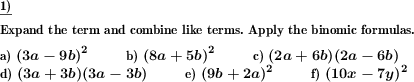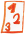Math worksheetsCustom math worksheets at your fingertips

# Details for problem "Expand and simplify binomic formula, advanced"

Quickname: 7517

## Summary

Apply binomic formula expansion rules to simplify term. Advanced version mit multiples of variables as summands.

## Example## Description

Apply the well known binomic formula expansion rules to expand and simplify terms. The summands of the formula's factors are multiples of a variable. The number of problems is selectable. For the types of formulas that will appear it can be specified that only some of the three binomic formulas will be used. Variables that appear will always be named a and b or x and y.

Download free worksheets for this math problem here. There is a second sheet with the solutions. Just click on the respective link.Worksheet 1Solution sheetWorksheet 2Solution sheetWorksheet 3Solution sheet

If you can not see the solution sheets for download, they may be filtered out by an ad blocker that you may have installed. If this is the case, please allow ads for this page and reload the page. The solution sheets will then reappear.

• Do the sample worksheets do not really fit?
• Do you need more math worksheets, with a different level of difficulty?
• Would you like to combine different problems on a worksheet and adjust them to your needs?
• As a teacher, you can put together your own worksheets using the automatically generated math problems provided.
With a free initial credit, you can start creating your own math worksheets in a few minutes.

It does not cost anything to try! Register here, to create custom worksheets now!

## Customization options for this problem

ParameterPossible values
Number of problems1, 2, 3, 4, 5, 6, 7, 8, 9, 10
Binomic formula types(a+b)^2, (a-b)^2, (a+b)(a-b), (a+b)^2 & (a-b)^2, all
Negative factors allowdYes, No